Video Tutorial Entropy Change

Quick Notes Entropy Change

• The standard entropy of a substance, So, refers to the entropy of 1 mole of the substance, measured at standard conditions (298K and 101kPa).
• Units for standard entropy are JK-1mol-1
• Entropy change, ΔS, for a reaction can be found using standard entropies of reacatants and products:
•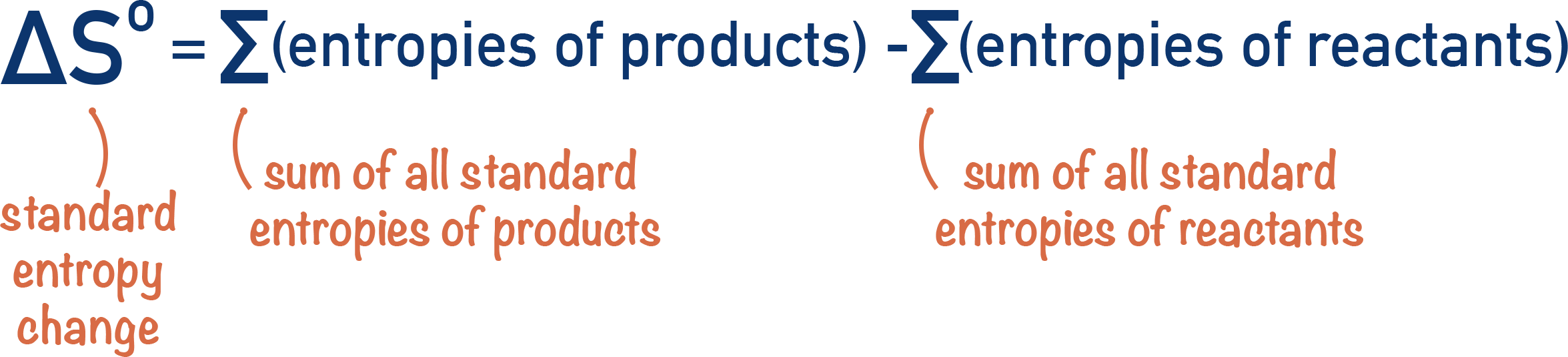• A substance has different entropies depending upon its state and conditions.
• Water has a low entropy below 0oC (as it’s a solid), but a high entropy at over 100oC (as it’s a gas).

Full Notes Entropy Change

Entropy is a way of measuring disorder, which means every substance has its own value of entropy as every substance is ‘ordered’ in a slightly different way.

For example...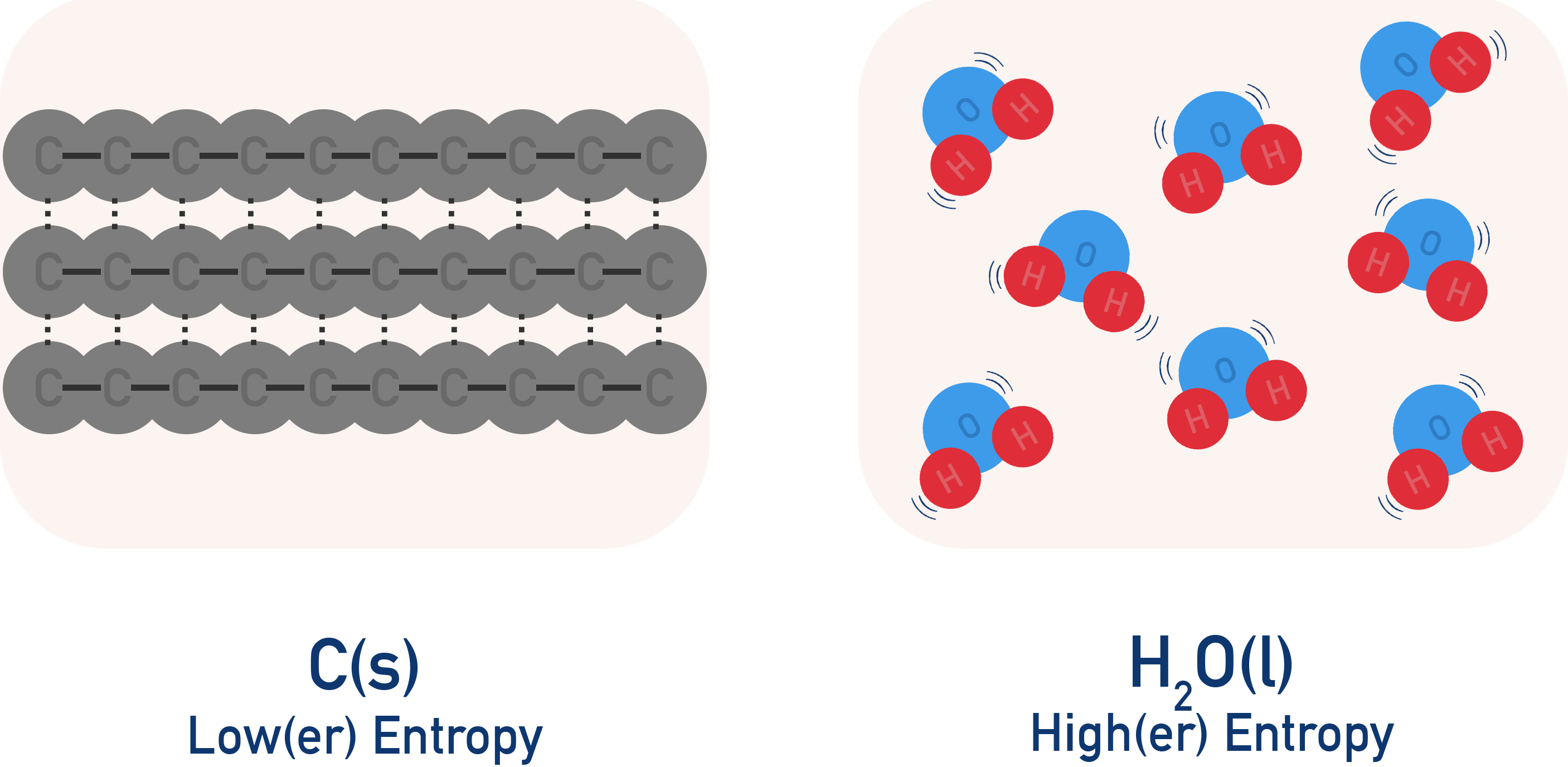The particles in solid carbon have a low entropy, as they are in a fixed position and have little room to move.

The molecules in a sample of liquid water, however, are not in a fixed position and can be very disordered, so liquid water has a high entropy.

Even if two substances are in the same state, they will still have different entropies as the particles in the solids will be arranged in slightly different ways. The ions in sodium chloride (NaCl) are packed together in a slightly different way to the ions in aluminium chloride (AlCl3). This means the level of disorder will be slightly different.

## Standard Entropies, So

The problem with describing a substance’s entropy is that entropy changes with temperature. Think about simple kinetic theory: the more energy you give a substance, the more the particles move. More movement of particles means more disorder, thus a higher level of entropy.

Solid water (ice) has a very low entropy; gaseous water (steam) has a very high entropy. In both cases the 'substance' is still water, made up of H2O molecules.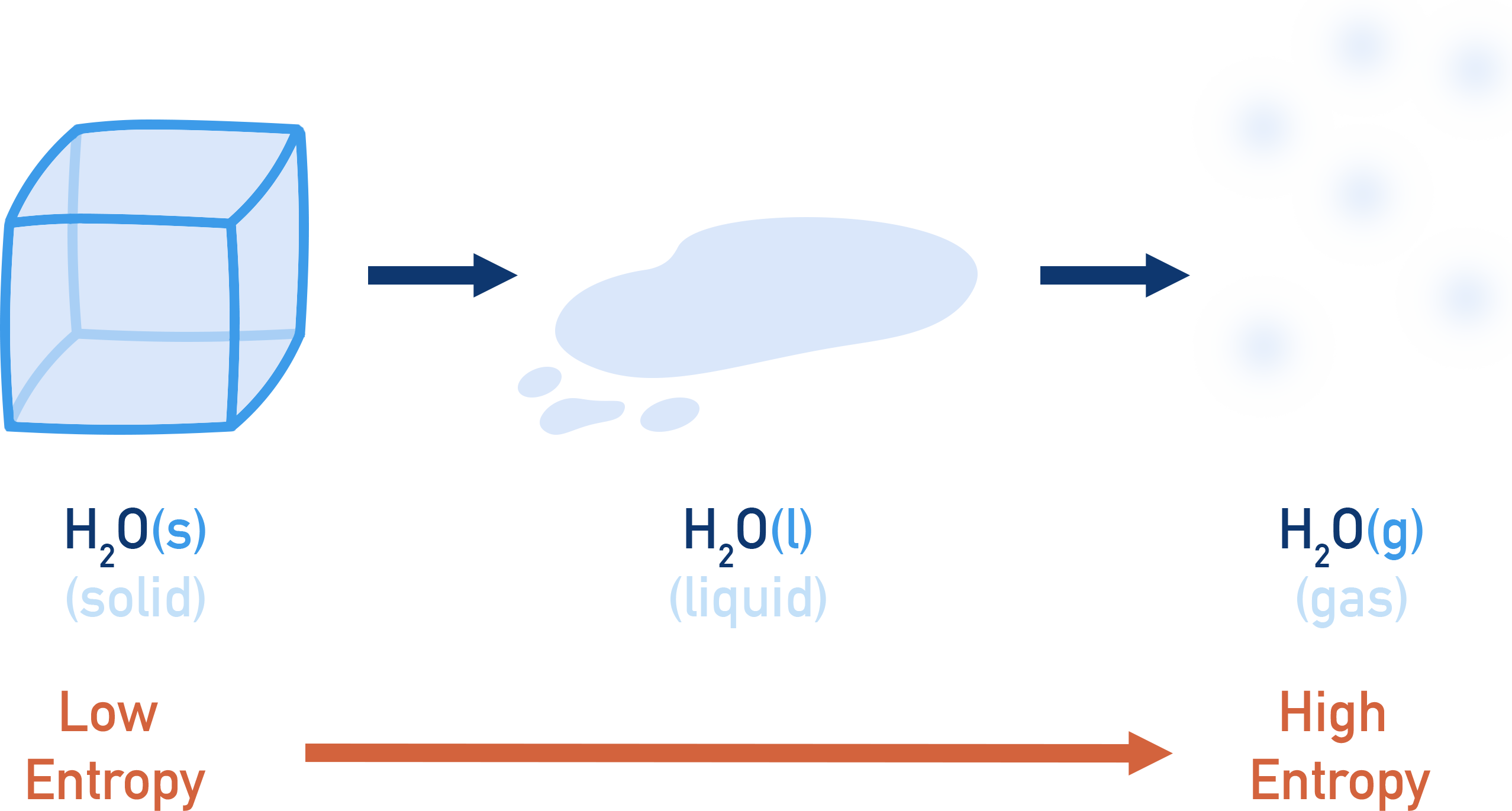The substance is the same chemically, but a change in its state has caused a change in entropy. This means if we want to compare the entropies of different substances, we need a ‘standard’ temperature and pressure to use for measurements. The conditions usually used are 298K and 101kPa. Values for entropies of a substance at these conditions are called 'standard entropies', shown as So.

For Example...

Under standard conditions, water is a liquid. The standard entropy of liquid water (H2O) refers to the entropy content of 1 mole of liquid water at 298 K and 101 kPa, ‘the standard entropy of H2O’.

## Entropy Change, ΔSo

As all substances have different entropies, when a reaction occurs the products formed will have different entropies to the reactants.This will mean the combined entropy of reactants will be different to the combined entropy of products and an 'overall' entropy change (ΔS) will occur.

The entropy change of a reaction can be predicted just by looking at the number of moles of reactants compared to products and their states.

For Example...

If the number of moles of particles increases in a reaction, that would imply an increase in entropy (+ΔS) as more particles gives a greater level of disorder.

The states of reactants and products is also important - if reactants are solids and the products formed are liquids or gases, this would give an increase in entropy (+ΔS). Equally, if liquids or gases react to form solids, this would give a decrease in entropy (-ΔS).

## Calculating entropy change (using standard entropies)

By using the standard entropies (So) of reactants and products, we can calculate the change in entropy for a reaction.

How much the entropy changes (ΔS) is based on the difference in the combined entropies of the reactants and products.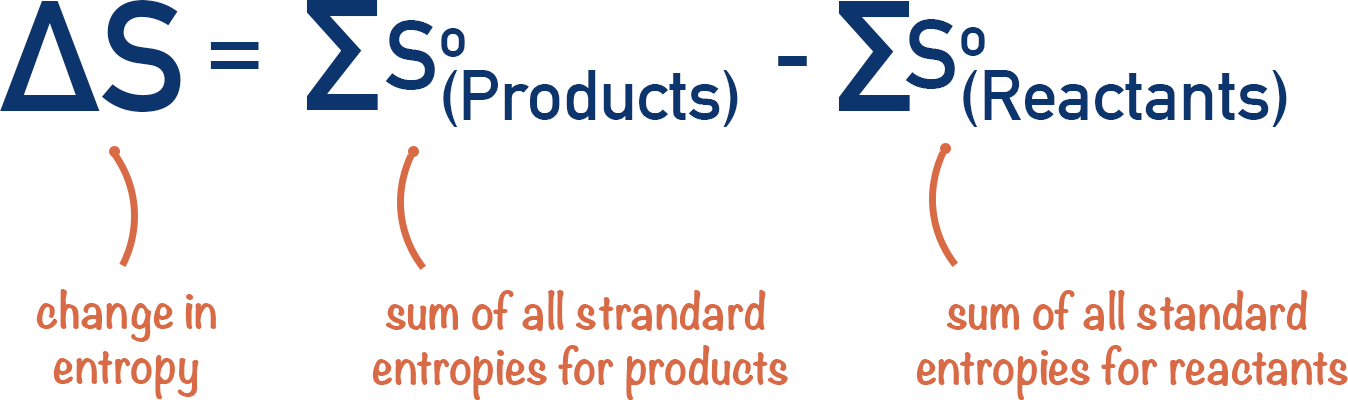For example...

Exam style question

Using the data given, calculate the entropy change for the following reaction.

CH4(g) + 2O2(g) → CO2(g) + 2H2O(l)

Species Standard Entropy, So(J K-1 mol-1)
CH4(g) 186.3
O2(g) 205.1
H2O(g) 69.9
CO2(g) 213.7

We need to find the combined standard entropies of reactants (CH4 and O2) and products (CO2 and H2O).

Note that the O2 and H2O entropy values have be multiplied by two as there are two moles worth of each in the reaction - entropy units are per mol.

Then subtract total entropy of reactants from products.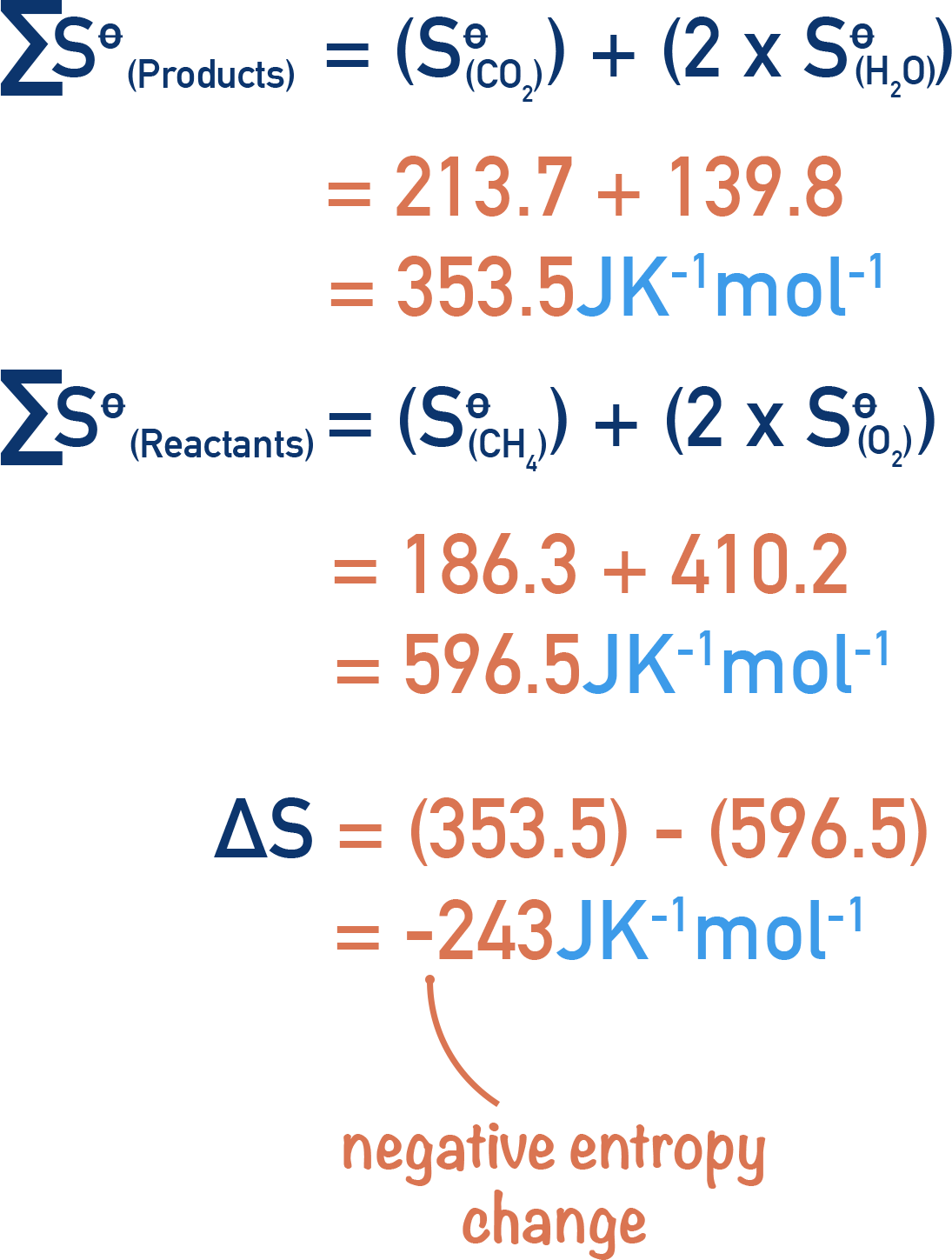One important thing to consider here is that standard entropies have units of J K-1 mol-1. This becomes important when using entropy values alongside enthalpy values (the standard units for enthalpies are kJ mol-1). See ‘Free Energy.